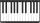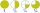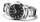# Fraction calculator

The calculator performs basic and advanced operations with fractions, expressions with fractions combined with integers, decimals, and mixed numbers. It also shows detailed step-by-step information about the fraction calculation procedure. Solve problems with two, three, or more fractions and numbers in one expression.

## Result:

### 1/2 : 2 = 1/4 = 0.25

Spelled result in words is one quarter.

### How do you solve fractions step by step?

1. Divide: 1/2 : 2 = 1/2 · 1/2 = 1 · 1/2 · 2 = 1/4
Dividing two fractions is the same as multiplying the first fraction by the reciprocal value of the second fraction. The first sub-step is to find the reciprocal (reverse the numerator and denominator, reciprocal of 2/1 is 1/2) of the second fraction. Next, multiply the two numerators. Then, multiply the two denominators. In the next intermediate step, the fraction result cannot be further simplified by canceling.
In words - one half divided by two = one quarter.

#### Rules for expressions with fractions:

Fractions - use the slash “/” between the numerator and denominator, i.e., for five-hundredths, enter 5/100. If you are using mixed numbers, be sure to leave a single space between the whole and fraction part.
The slash separates the numerator (number above a fraction line) and denominator (number below).

Mixed numerals (mixed fractions or mixed numbers) write as non-zero integer separated by one space and fraction i.e., 1 2/3 (having the same sign). An example of a negative mixed fraction: -5 1/2.
Because slash is both signs for fraction line and division, we recommended use colon (:) as the operator of division fractions i.e., 1/2 : 3.

Decimals (decimal numbers) enter with a decimal point . and they are automatically converted to fractions - i.e. 1.45.

The colon : and slash / is the symbol of division. Can be used to divide mixed numbers 1 2/3 : 4 3/8 or can be used for write complex fractions i.e. 1/2 : 1/3.
An asterisk * or × is the symbol for multiplication.
Plus + is addition, minus sign - is subtraction and ()[] is mathematical parentheses.
The exponentiation/power symbol is ^ - for example: (7/8-4/5)^2 = (7/8-4/5)2

#### Examples:

subtracting fractions: 2/3 - 1/2
multiplying fractions: 7/8 * 3/9
dividing Fractions: 1/2 : 3/4
exponentiation of fraction: 3/5^3
fractional exponents: 16 ^ 1/2
adding fractions and mixed numbers: 8/5 + 6 2/7
dividing integer and fraction: 5 ÷ 1/2
complex fractions: 5/8 : 2 2/3
decimal to fraction: 0.625
Fraction to Decimal: 1/4
Fraction to Percent: 1/8 %
comparing fractions: 1/4 2/3
multiplying a fraction by a whole number: 6 * 3/4
square root of a fraction: sqrt(1/16)
reducing or simplifying the fraction (simplification) - dividing the numerator and denominator of a fraction by the same non-zero number - equivalent fraction: 4/22
expression with brackets: 1/3 * (1/2 - 3 3/8)
compound fraction: 3/4 of 5/7
fractions multiple: 2/3 of 3/5
divide to find the quotient: 3/5 ÷ 2/3

The calculator follows well-known rules for order of operations. The most common mnemonics for remembering this order of operations are:
PEMDAS - Parentheses, Exponents, Multiplication, Division, Addition, Subtraction.
BEDMAS - Brackets, Exponents, Division, Multiplication, Addition, Subtraction
BODMAS - Brackets, Of or Order, Division, Multiplication, Addition, Subtraction.
GEMDAS - Grouping Symbols - brackets (){}, Exponents, Multiplication, Division, Addition, Subtraction.
Be careful, always do multiplication and division before addition and subtraction. Some operators (+ and -) and (* and /) has the same priority and then must evaluate from left to right.

## Fractions in word problems:

• Larry 2Larry spends half of his workday teaching piano lessons. If he sees 6 students, each for the same amount of time, what fraction of his workday is spent with each student?
• AlmondsRudi has 4 cups of almonds. His trail mix recipe calls for 2/3 cup of almonds. How many batches of trail mix can he make?
• Two dividedTwo divided by nine-tenths.
• Mario 2Mario is preparing a dinner for his friends. He made 3 bowls of spaghetti sauce. If each bowl holds 3/4 quart, how much sauce did Mario make?
• JonahJonah has an 8-pound bag of potting soil. He divides it evenly among 5 flowerpots. How much soil is in each pot? Show your answer as a fraction or mixed number. Solve this problem any way you choose.
• MinutesWrite as fraction in basic form which part of the week is 980 minutes.
• Evaluate expressionEvaluate expression using BODMAS rule: 1 1/4+1 1/5÷3/5-5/8
• DicesWe will throw two dice. What is the probability that the ratio between numbers on first and second dice will be 1:2?
• The thirdThe one-third rod is blue, one-half of the rod is red, the rest of the rod is white and measures 8 cm. How long is the whole rod?
• Gear wheelsTwo gear wheels, which fit together, have the number of teeth z1=58 and z2=149. Calculate the speed of the first wheel, if the second wheel rotates 1232 revolutions per minute.
• Ornamental shrubsChildren committed to planting 240 ornamental shrubs. Their commitment, however, exceeded by 48 shrubs. Write the ratio of actually planted shrubs and commitment by lowest possible integers a/b.
• PediatricianPediatrician this month of 20 working days takes 8 days holidays. What is the probability that on Monday it will be at work?
• Drinking waterA man drinks a keg of water in 40 days, and a woman drinks in 62 days. How many days do they consume a keg together?